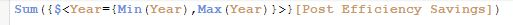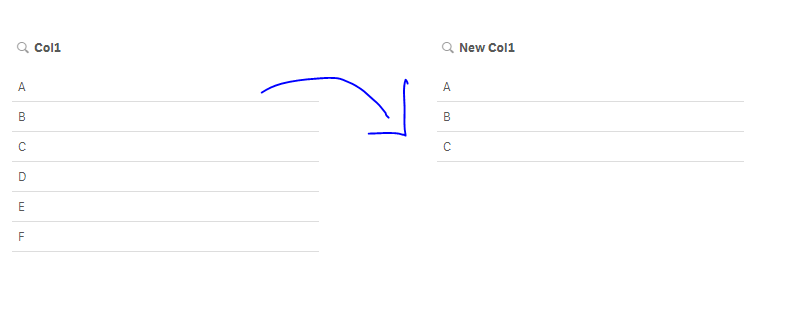New to Qlik Sense

Discussion board where members can get started with Qlik Sense.

Contributor

Using set analysis/filters for dimensions

I have this table:

Col1    Col2

A            12

B            9

C            2

D            5

E            6

F            10

I'm building a bar chart and I need Col1 as the dimension. But I need only values B, C, E, F (say).

How do we use set analysis or filters here?

If we're using filters, the user should not have the freedom to manipulate this selection. At the same time, I need all the values available for other graphs in the sheet.

Tags (2)
1 Solution

Accepted SolutionsMVP

Re: Using set analysis/filters for dimensions

May be this

Sum({<Col1 = {'B', 'C', 'E', 'F'}>}Col2)

7 RepliesPartner

Re: Using set analysis/filters for dimensions

use set analysis in chart expression like below:

ex. sum( {<  Col1 = {'B','C','E','F'} >} Col2 )MVP

Re: Using set analysis/filters for dimensions

May be this

Sum({<Col1 = {'B', 'C', 'E', 'F'}>}Col2)

Contributor

Re: Using set analysis/filters for dimensions

Thanks. Can you tell me why this code isn't working. I seem to be missing something really simple here.MVP

Re: Using set analysis/filters for dimensions

Missing a dollar sign expansion

Sum({<Year = {\$(=Min(Year)), \$(=Max(Year))}>} [Post Efficiency Savings])Partner

Re: Using set analysis/filters for dimensions

If you want to have a filter that contains only certain values of the col1, you can create a master dimension as follow:

=if( match(Col1,'A','B','C'), Col1,Null())

and then add it as a filter :Highlighted
Contributor

Re: Using set analysis/filters for dimensions

Thanks Omar. Really helpful.

Contributor

Re: Using set analysis/filters for dimensions

Thanks a lot Sunny.